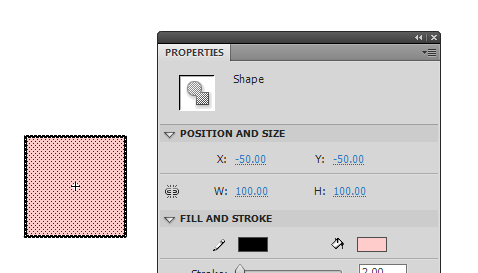# Changing a Movieclip registration point on the fly with AS3

Do you know what is a Movieclip registration point? If you ever played with Flash drawing tools, you should.

The registration point is like the origin in a Cartesian coordinate system, the default point of a Movieclip from which its x and y coordinates are calculated.

In the example we are about to see, I created this square with a 100 pixel side and placed at (-50,-50), so its center of rotation is at (0,0), as you can see from the tiny white crosshair in the centre of the square.Now the question is: can I change dynamically the registration point with AS3?

Anyway, there is a little trick.

Look at this script:

```package {
import flash.display.Sprite;
public class registration extends Sprite {
private var square:square_mc;
private var static_square:static_square_mc
public function registration() {
square = new square_mc(100,100);
square.x = 100
square.y = 100
static_square = new static_square_mc()
static_square.x = 100
static_square.y = 100
static_square.alpha=0.3
//
square = new square_mc(50,50);
square.x = 250
square.y = 100
static_square = new static_square_mc()
static_square.x = 250
static_square.y = 100
static_square.alpha=0.3
//
square = new square_mc(0,0);
square.x = 400
square.y = 100
static_square = new static_square_mc()
static_square.x = 400
static_square.y = 100
static_square.alpha=0.3
}
}
}```

In a very lazy way (because it’s not the focus of this post) it creates three squares and three static squares.

Let’s concentrate on `square_mc` class constructor:

`square = new square_mc(x,y);`

Where `x` and `y` represent the coordinates of the new registration point. So (0,0) will mean the upper left corner, (50,50) the center and (100,100) the lower right corner.

Let’s see `square_mc.as`

```package {
import flash.display.Sprite;
import flash.events.Event;
import flash.geom.Rectangle;
public class square_mc extends Sprite {
private var new_reg_x,new_reg_y:int;
private var pin:pin_mc=new pin_mc();
public function square_mc(reg_x,reg_y) {
new_reg_x=reg_x;
new_reg_y=reg_y;
}
var rect:Rectangle=getBounds(this);
var x_offset=new_reg_x+rect.x;
var y_offset=new_reg_y+rect.y
// updating container position
x+=x_offset;
y+=y_offset;
// just adding a pin to highlight registration point
var par:registration=this.parent as registration
pin.x=x;
pin.y=y
for (var i:uint=0; i
First, I get the bounds rectangle of the object (not necessary since I know it's a square, but I could be anything else), then I determine the offset adding to new registration coordinates the origin of the bounds rectangle. This will work because I drew the square centered on movieclip origin.
Once I found the offset, it's just a matter of adding the offset to square position and subtracting from all its children's (the square shape) position.
And that's it:

Obviously you can set the new registration point anywhere you want, and it will work anyway.
Just a quick question: what game prototype I am going to show you using this feature?
```
214 GAME PROTOTYPES EXPLAINED WITH SOURCE CODE
// 1+2=3
// 10000000
// 2 Cars
// 2048
// Avoider
// Ballz
// Block it
// Blockage
// Bloons
// Boids
// Bombuzal
// Breakout
// Bricks
// Columns
// CubesOut
// Dots
// DROP'd
// Dudeski
// Eskiv
// Filler
// Fling
// Globe
// HookPod
// Hundreds
// InkTd
// Iromeku
// Lumines
// Magick
// MagOrMin
// Maze
// Memdot
// Nano War
// Nodes
// o:anquan
// Ononmin
// Pacco
// Phyballs
// Platform
// Poker
// Pool
// Poux
// Pudi
// qomp
// Racing
// Renju
// SameGame
// Security
// Sling
// Slingy
// Sokoban
// Splitter
// Sproing
// Stack
// Stringy
// Sudoku
// Tetris
// Threes
// Toony
// Turn
// TwinSpin
// vvvvvv
// Wordle
// Worms
// Yanga
// Zhed
// zNumbers